轻量函数式 JavaScript：九、递归

+关注继续查看

(本页的剩余部分故意被留作空白)

定义

function foo(x) {
if (x < 5) return x;
return foo( x / 2 );
}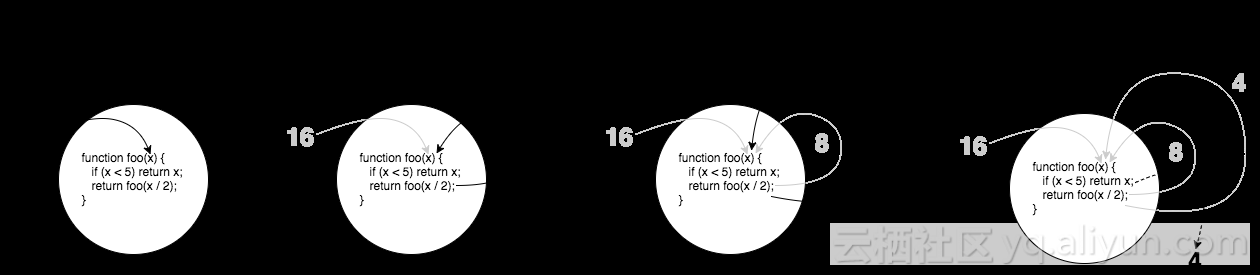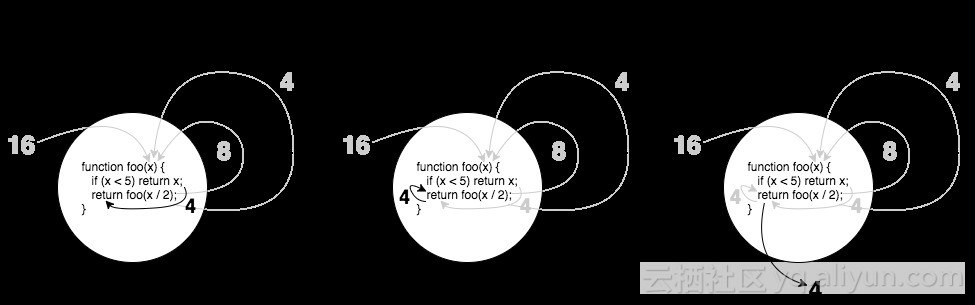function isPrime(num,divisor = 2){
if (num < 2 || (num > 2 && num % divisor == 0)) {
return false;
}
if (divisor <= Math.sqrt( num )) {
return isPrime( num, divisor + 1 );
}

return true;
}

fib( 0 ): 0
fib( 1 ): 1
fib( n ):
fib( n - 2 ) + fib( n - 1 )

function fib(n) {
if (n <= 1) return n;
return fib( n - 2 ) + fib( n - 1 );
}

fib(..) 递归地调用自己两次，这通常被称为二元递归。我们稍后将会更多地谈到二元递归。

相互递归

function isOdd(v) {
if (v === 0) return false;
return isEven( Math.abs( v ) - 1 );
}

function isEven(v) {
if (v === 0) return true;
return isOdd( Math.abs( v ) - 1 );
}

function fib_(n) {
if (n == 1) return 1;
else return fib( n - 2 );
}

function fib(n) {
if (n == 0) return 0;
else return fib( n - 1 ) + fib_( n );
}

为什么要递归？

function sum(total,...nums) {
for (let i = 0; i < nums.length; i++) {
total = total + nums[i];
}

}

// vs

function sum(num1,...nums) {
if (nums.length == 0) return num1;
return num1 + sum( ...nums );
}

声明式递归

function maxEven(...nums) {
var num = -Infinity;

for (let i = 0; i < nums.length; i++) {
if (nums[i] % 2 == 0 && nums[i] > num) {
num = nums[i];
}
}

if (num !== -Infinity) {
return num;
}
}

maxEven( nums ):
maxEven( nums.0, maxEven( ...nums.1 ) )

maxEven( 1, 10, 3, 2 ):
maxEven( 1, maxEven( 10, maxEven( 3, maxEven( 2 ) ) )

function maxEven(num1,...restNums) {
var maxRest = restNums.length > 0 ?
maxEven( ...restNums ) :
undefined;

return (num1 % 2 != 0 || num1 < maxRest) ?
maxRest :
num1;
}

maxEven( num1, ...restNums ):
maxEven( num1, maxEven( ...restNums ) )

depth( node ):
1 + max( depth( node.left ), depth( node.right ) )

function depth(node) {
if (node) {
let depthLeft = depth( node.left );
let depthRight = depth( node.right );
return 1 + max( depthLeft, depthRight );
}

return 0;
}

栈

function isOdd(v) {
if (v === 0) return false;
return isEven( Math.abs( v ) - 1 );
}

function isEven(v) {
if (v === 0) return true;
return isOdd( Math.abs( v ) - 1 );
}

isOdd( 33333 );            // RangeError: Maximum call stack size exceeded

function foo() {
var z = "foo!";
}

function bar() {
var y = "bar!";
foo();
}

function baz() {
var x = "baz!";
bar();
}

baz();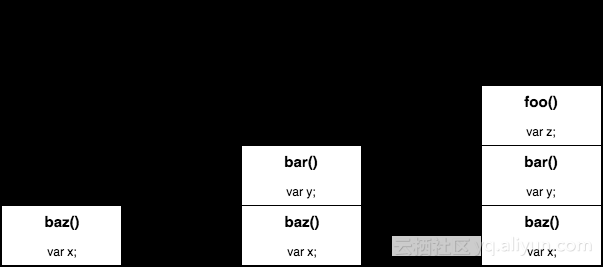尾部调用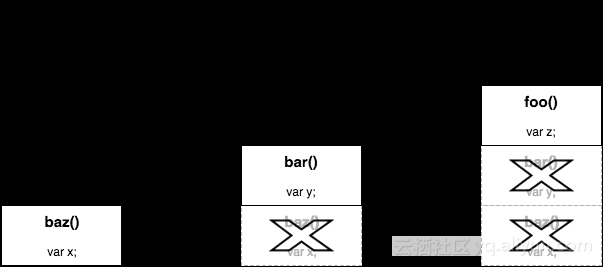正确尾部调用（PTC）

return foo( .. );

foo();
return;

// 或者

var x = foo( .. );
return x;

// 或者

return 1 + foo( .. );

1 + 的那一部分绝对会在 foo(..) 完成 之后 处理，所以栈帧不得不保留。

return x ? foo( .. ) : bar( .. );

重新安排递归

替换栈

function sum(num1,...nums) {
if (nums.length == 0) return num1;
return num1 + sum( ...nums );
}

function sum(result,num1,...nums) {
// ..
}

"use strict";

function sum(result,num1,...nums) {
result = result + num1;
if (nums.length == 0) return result;
return sum( result, ...nums );
}

sum( /*initialResult=*/0, 3, 1, 17, 94, 8 );        // 123

"use strict";

function sumRec(result,num1,...nums) {
result = result + num1;
if (nums.length == 0) return result;
return sumRec( result, ...nums );
}

function sum(...nums) {
return sumRec( /*initialResult=*/0, ...nums );
}

sum( 3, 1, 17, 94, 8 );                                // 123

"use strict";

function sum(...nums) {
return sumRec( /*initialResult=*/0, ...nums );

function sumRec(result,num1,...nums) {
result = result + num1;
if (nums.length == 0) return result;
return sumRec( result, ...nums );
}
}

sum( 3, 1, 17, 94, 8 );                                // 123

"use strict";

var sum = (function IIFE(){

return function sum(...nums) {
return sumRec( /*initialResult=*/0, ...nums );
}

function sumRec(result,num1,...nums) {
result = result + num1;
if (nums.length == 0) return result;
return sumRec( result, ...nums );
}

})();

sum( 3, 1, 17, 94, 8 );                                // 123

"use strict";

function sum(result,num1,...nums) {
result = result + num1;
if (nums.length == 0) return result;
return sum( result, ...nums );
}

sum( /*initialResult=*/0, 3, 1, 17, 94, 8 );        // 123

"use strict";

function sum(num1,num2,...nums) {
num1 = num1 + num2;
if (nums.length == 0) return num1;
return sum( num1, ...nums );
}

sum( 3, 1, 17, 94, 8 );                                // 123

1. 一开始先比较头两个数字，num1num2
2. num1 是偶数，而且 num1 大于 num2 吗？如果是，保持 num1
3. 如果 num2 是偶数，保持它（存储在 num1 中）。
4. 否则。退回到 undefined（存储在 num1 中）。
5. 如果有更多的 nums 要考虑，将它们递归地与 num1 进行比较。
6. 最后，返回留在 num1 中的任何值。

"use strict";

function maxEven(num1,num2,...nums) {
num1 =
(num1 % 2 == 0 && !(maxEven( num2 ) > num1)) ?
num1 :
(num2 % 2 == 0 ? num2 : undefined);

return nums.length == 0 ?
num1 :
maxEven( num1, ...nums )
}

延续传递风格（CPS）

"use strict";

function fib(n,cont = identity) {
if (n <= 1) return cont( n );
return fib(
n - 2,
n2 => fib(
n - 1,
n1 => cont( n2 + n1 )
)
);
}

蹦床

CPS 创建延续并传递它们，另一种减轻内存压力的技术称为蹦床（trampolines）。在这种风格的代码中，会创建 CPS 形式的延续，但它们不是被传入，而是被浅层地返回。

function trampoline(fn) {
return function trampolined(...args) {
var result = fn( ...args );

while (typeof result == "function") {
result = result();
}

return result;
};
}

var sum = trampoline(
function sum(num1,num2,...nums) {
num1 = num1 + num2;
if (nums.length == 0) return num1;
return () => sum( num1, ...nums );
}
);

var xs = [];
for (let i=0; i<20000; i++) {
xs.push( i );
}

sum( ...xs );                    // 199990000

总结10095 0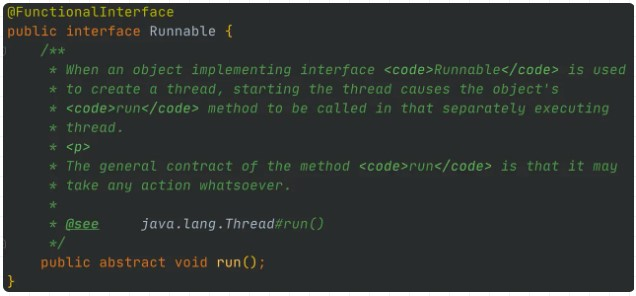Java8的函数式接口你真的了解吗？

1169 0在博客动态规划法（一）从斐波那契数列谈起中，在求解斐波那契数列的第n项时，我们采用了递归方法和动态规划法来求解，当然递归方法的效率很差。
1195 0Java经典类库-Guava中的函数式编程讲解

779 013893 0JavaScript创建对象（四）——组合使用构造函数和原型模式

825 0903 0C++程序设计-第13周递归函数及银行系统程序设计上机实践项目

1137 0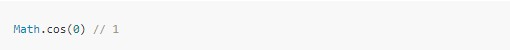336 0JavaScript创建对象（二）——构造函数模式

841 0

12

0

《2021云上架构与运维峰会演讲合集》

《零基础CSS入门教程》

《零基础HTML入门教程》